# 9 Results

View
Selected filters:
• Numbers to 1000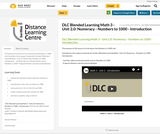Conditional Remix & Share Permitted
CC BY-NC-SA
Rating

The purpose of this lesson is to introduce the Numbers to 1000 unit.

Included are the support materials for Grade 3 Blended Learning Math - Unit 2.0: Patterning -Numbers to 1000 - Introduction:
- YouTube video on introducing the unit on Numbers to 1000

Subject:
Math
Material Type:
Activity/Lab
Homework/Assignment
Lesson
Provider:
Sun West Distance Learning Centre (DLC)
Author:
Sun West School Division
04/04/2019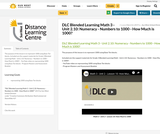Conditional Remix & Share Permitted
CC BY-NC-SA
Rating

The purpose of this lesson is to represent 1000 using Base Ten blocks.

Included are the support materials for Grade 3 Blended Learning Math - Unit 2.10: Numeracy - Numbers to 1000 - How Much is 1000?:
- YouTube video on representing 1000 using Base Ten blocks

Subject:
Math
Material Type:
Activity/Lab
Homework/Assignment
Lesson
Provider:
Sun West Distance Learning Centre (DLC)
Author:
Sun West School Division
04/04/2019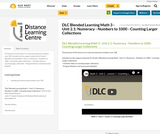Conditional Remix & Share Permitted
CC BY-NC-SA
Rating

The purpose of this lesson is to read and represent numbers over 100.

Included are the support materials for Grade 3 Blended Learning Math - Unit 2.1: Numeracy - Numbers to 1000 - Counting Larger Collections:
- YouTube video on counting larger collections

Subject:
Math
Material Type:
Activity/Lab
Homework/Assignment
Lesson
Provider:
Sun West Distance Learning Centre (DLC)
Author:
Sun West School Division
04/04/2019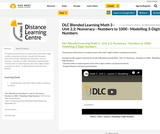Conditional Remix & Share Permitted
CC BY-NC-SA
Rating

The purpose of this lesson is to explore place value with 3-digit numbers using base ten blocks.

Included are the support materials for Grade 3 Blended Learning Math - Unit 2.2: Numeracy - Numbers to 1000 - Modelling 3-Digit Numbers:
- YouTube video on exploring place value with 3-digit numbers using base ten blocks

Subject:
Math
Material Type:
Activity/Lab
Homework/Assignment
Lesson
Provider:
Sun West Distance Learning Centre (DLC)
Author:
Sun West School Division
04/04/2019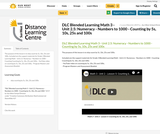Conditional Remix & Share Permitted
CC BY-NC-SA
Rating

The purpose of this lesson is to skip count by 5s, 10s, 25s and 100s.

Included are the support materials for Grade 3 Blended Learning Math - Unit 2.5: Numeracy - Numbers to 1000 - Counting Counting by 5s, 10s, 25s and 100s:
- YouTube video on counting by 5s, 10s, 25s and 100s,

Subject:
Math
Material Type:
Activity/Lab
Homework/Assignment
Lesson
Provider:
Sun West Distance Learning Centre (DLC)
Author:
Sun West School Division
04/04/2019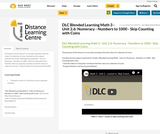Conditional Remix & Share Permitted
CC BY-NC-SA
Rating

The purpose of this lesson is to use skip counting to find the value of a collection of coins.

Included are the support materials for Grade 3 Blended Learning Math - Unit 2.6: Numeracy - Numbers to 1000 - Skip Counting with Coins:
- YouTube video on using skip counting to find the value of a collection of coins

Subject:
Math
Material Type:
Activity/Lab
Homework/Assignment
Lesson
Provider:
Sun West Distance Learning Centre (DLC)
Author:
Sun West School Division
04/04/2019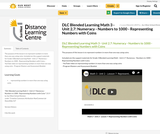Conditional Remix & Share Permitted
CC BY-NC-SA
Rating

The purpose of this lesson is to represent numbers in more than one way using coins.

Included are the support materials for Grade 3 Blended Learning Math - Unit 2.7: Numeracy - Numbers to 1000 - Representing Numbers with Coins:
- YouTube video on representing numbers in more than one way using coins

Subject:
Math
Material Type:
Activity/Lab
Homework/Assignment
Lesson
Provider:
Sun West Distance Learning Centre (DLC)
Author:
Sun West School Division
04/04/2019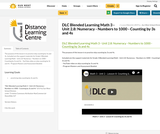Conditional Remix & Share Permitted
CC BY-NC-SA
Rating

The purpose of this lesson is to practice skip counting by 3s and 4s.

Included are the support materials for Grade 3 Blended Learning Math - Unit 2.8: Numeracy - Numbers to 1000 - Counting by 3s and 4s:
- YouTube video on skip counting by 3s and 4s

Subject:
Math
Material Type:
Activity/Lab
Homework/Assignment
Lesson
Provider:
Sun West Distance Learning Centre (DLC)
Author:
Sun West School Division
04/04/2019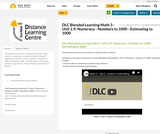Conditional Remix & Share Permitted
CC BY-NC-SA
Rating

The purpose of this lesson is to estimate a quantity using a referent.

Included are the support materials for Grade 3 Blended Learning Math - Unit 2.9: Numeracy - Numbers to 1000 - Estimating to 1000:

Subject:
Math
Material Type:
Activity/Lab
Homework/Assignment
Lesson
Provider:
Sun West Distance Learning Centre (DLC)
Author:
Sun West School Division